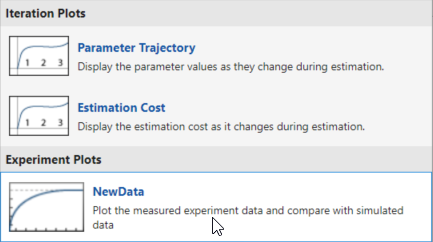## Plot and Analyze Data

### Why Plot the Data Before Parameter Estimation

After you import the estimation data as described in Import Data for Parameter Estimation, you can remove outliers, smooth, detrend, or otherwise treat the data to prepare for analysis and estimation. To view and analyze data characteristics, plot the data on a time plot.

### Plot Data

Use an experiment plot to visualize experiment data. First, create an experiment and import data as described in Import Data for Parameter Estimation. To create an experiment plot, in the Parameter Estimator, on the Parameter Estimation tab, click Add Plot, and select NewData under Experiment Plots.This creates plots of the input signal for the Inport block `BPAV` and output signal for the Outport block ```Engine Speed``` for the `engine_idle_speed` model (see Create Experiment).You can also plot the experiment data by right-clicking `NewData` and selecting Plot measured experiment data from the list.

Using the time plot, you can examine the data characteristics such as noise, outliers and portions of the data to use for estimating parameters. After you analyze the data, you can preprocess it as described in Preprocess Data.